## Example Questions

### Example Question #1 : Simplifying Fractions

Simplify x/2 – x/5

3x/10

3x/7

7x/10

2x/7

5x/3

3x/10

Explanation:

Simplifying this expression is similar to 1/2 – 1/5.  The denominators are relatively prime (have no common factors) so the least common denominator (LCD) is 2 * 5 = 10.  So the problem becomes 1/2 – 1/5 = 5/10 – 2/10 = 3/10.

### Example Question #2 : Simplifying Fractions

If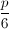is an integer, which of the following is a possible value of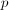?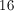Explanation: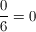, which is an integer (a number with no fraction or decimal part).  All the other choices reduce to non-integers.

### Example Question #3 : Simplifying Fractions

Simplify: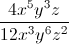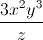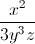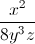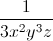Explanation: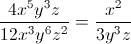First, let's simplify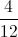. The greatest common factor of 4 and 12 is 4. 4 divided by 4 is 1 and 12 divided by 4 is 3. Therefore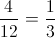.

To simply fractions with exponents, subtract the exponent in the numerator from the exponent in the denominator. That leaves us with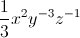or### Example Question #4 : Simplifying Fractions

Which of the following is not equal to 32/24?

16/12

224/168

4/3

160/96

96/72

160/96

Explanation:

24/32 = 1.33

16/12 =1.33

224/168 =1.33

4/3 = 1.33

96/72 = 1.33

160/96 = 1.67

### Example Question #5 : Simplifying Fractions

Find the root ofCan not be determined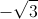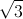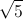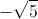Explanation: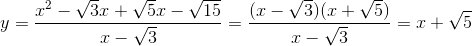The root occurs where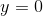. So we substitute 0 for.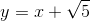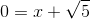This means that the root is at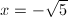.

### Example Question #6 : Simplifying Fractions

Simplify the fraction below: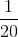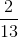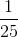Explanation:

The correct approach to solve this problem is to first write factors for the numerator and the denominator: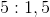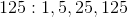The highest common factor is 5. Therefore, you can divide the numerator and denominator by 5 in order to get a simplified fraction.

Thus the numerator becomes,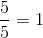and the denominator becomes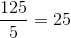.

Therefore the final answer is.

### Example Question #7 : Simplifying Fractions

Simplify: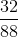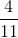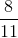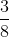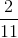Explanation:

Find the common factors of the numerator and denominator.  They both share factors of 2,4, and 8.  For simplicity, factor out an 8 from both terms and simplify.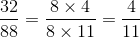### Example Question #8 : Simplifying Fractions

Simply the following fraction: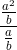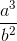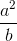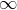Explanation:

Remember that when you divide a fraction by a fraction, that is the same as multiplying the fraction in the numerator by the reciprocal of the fraction in the denominator.

In other words,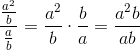Simplifying this final fraction gives us our correct answer,.

### Example Question #9 : Simplifying Fractions

Solve for.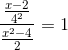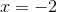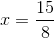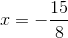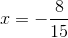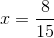Explanation:

To solve for, simplify the fraction. In order to do this, recall that dividing by a fraction is the same as multiplying by its reciprocal. Therefore, rewrite the equation as follows.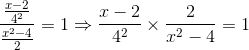Now, simplify the first fraction by calculating four squared.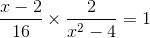From here, factor the denominator of the second fraction.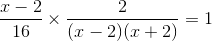Next, factor the 16.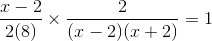From here, cancel out like terms that are in both the numerator and denominator. In this particular case that includes (x-2) and 2.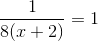Now, distribute the eight.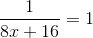Next, multiply both sides by the denominator.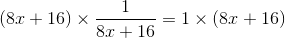The (8x+16) cancels out and leaves the following equation.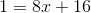Now to solve forperform opposite operations to move all numerical values to one side of the equation leavingby itself on the other side of the equation.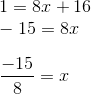### Example Question #10 : Simplifying Fractions

Which of the following fractions is not equivalent to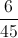?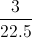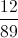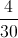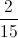Explanation:

Let us simplify: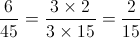We can get alternate forms of the same fraction by multiplying the denominator and the numerator by the same number: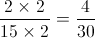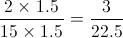Now let's look at: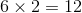, but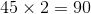.

Therefore,is the correct answer, as it is not equivalent to.